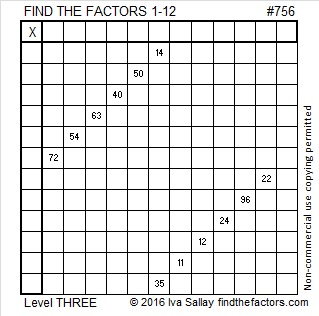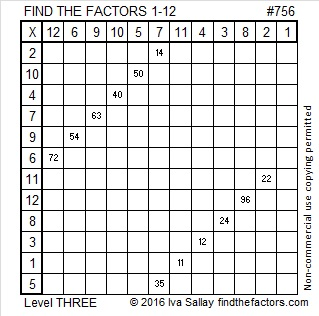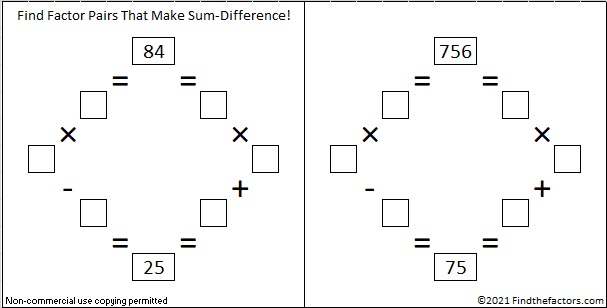# 756 and Level 3

Contents

### Today’s Puzzle:Print the puzzles or type the solution on this excel file: 12 Factors 2016-01-25

A Logical Approach to solve a FIND THE FACTORS puzzle: Find the column or row with two clues and find their common factor. (None of the factors are greater than 12.)  Write the corresponding factors in the factor column (1st column) and factor row (top row).  Because this is a level three puzzle, you have now written a factor at the top of the factor column. Continue to work from the top of the factor column to the bottom, finding factors and filling in the factor column and the factor row one cell at a time as you go.### Factor Trees for 756:

756 has many factors and, therefore, it has many possible factor trees. Here are three of them:### Factors of 756:

• 756 is a composite number.
• Prime factorization: 756 = 2 x 2 x 3 x 3 x 3 x 7, which can be written 756 = 2² x 3³ x 7
• The exponents in the prime factorization are 2, 3 and 1. Adding one to each and multiplying we get (2 + 1)(3 + 1)(1 + 1) = 3 x 4 x 2 = 24. Therefore 756 has exactly 24 factors.
• Factors of 756: 1, 2, 3, 4, 6, 7, 9, 12, 14, 18, 21, 27, 28, 36, 42, 54, 63, 84, 108, 126, 189, 252, 378, 756
• Factor pairs: 756 = 1 x 756, 2 x 378, 3 x 252, 4 x 189, 6 x 126, 7 x 108, 9 x 84, 12 x 63, 14 x 54, 18 x 42, 21 x 36 or 27 x 28
• Taking the factor pair with the largest square number factor, we get √756 = (√21)(√36) = 6√21 ≈ 27.495454.### Sum-Difference Puzzles:

84 has six factor pairs. One of those pairs adds up to 25, and another one subtracts to 25. Put the factors in the appropriate boxes in the first puzzle.

756 has twelve factor pairs. One of the factor pairs adds up to ­75, and a different one subtracts to 75. If you can identify those factor pairs, then you can solve the second puzzle!The second puzzle is really just the first puzzle in disguise. Why would I say that?

### More about the Number 756:

The last two digits of 756 is divisible by 4 so 756 is divisible by 4.

756 is formed from 3 consecutive numbers (5, 6, 7) so it is divisible by 3. The middle number is divisible by 3 so 756 is also divisible by 9.

756 can be written as the sum of consecutive numbers seven ways:

• 251 + 252 + 253 = 756; that’s 3 consecutive numbers.
• 105 + 106 + 107 + 108 + 109 + 110 + 111 = 756; that’s 7 consecutive numbers.
• 91 + 92 + 93 + 94 + 95 + 96 + 97 + 98 = 756; that’s 8 consecutive numbers.
• 80 + 81 + 82 + 83 + 84 + 85 + 86 + 87 + 88 = 756; that’s 9 consecutive numbers.
• 26 + 27 + 28 + 29 + 30 + 31 + 32 + 33 + 34 + 35 + 36 + 37 + 38 + 39 + 40 + 41 + 42 + 43 + 44 + 45 + 46 = 756; that’s 21 consecutive numbers.
• 20 + 21 + 22 + 23 + 24 + 25 + 26 + 27 + 28 + 29 + 30 + 31 + 32 + 33 + 34 + 35 + 36 + 37 + 38 + 39 + 40 + 41 + 42 + 43 = 756; that’s 24 consecutive numbers.
• 15 + 16 + 17 + 18 + 19 + 20 + 21 + 22 + 23 + 24 + 25 + 26 + 27 + 28 + 29 + 30 + 31 + 32 + 33 + 34 + 35 + 36 + 37 + 38 + 39 + 40 + 41  = 756; that’s 27 consecutive numbers.

756 is also the sum of six consecutive prime numbers: 109 + 113 + 127+ 131 + 137+ 139 = 756.

756 can be written as the sum of three squares four different ways. (Notice that all of the squares are even):

• 26² + 8² + 4² = 756
• 24² + 12² + 6² = 756
• 22² + 16² + 4² = 756
• 20² + 16² + 10² = 756

756 is a palindrome in two other bases:

• 11011 BASE 5; note that 1(625) + 1(125) + 0(25) + 1(5) + 1(1) = 756.
• LL BASE 35 (L is 21 base 10); note that 21(35) + 21(1) = 756.

This site uses Akismet to reduce spam. Learn how your comment data is processed.